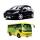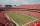# Two numbers

The sum of two numbers is 1. Identify this two numbers if you know that the half of first is equal to the third of second number.

Result

a =  0.4
b =  0.6

#### Solution:

a+b=1
a/2 = b/3

a+b = 1
3a-2b = 0

a = 25 = 0.4
b = 35 = 0.6

Calculated by our linear equations calculator.

Leave us a comment of example and its solution (i.e. if it is still somewhat unclear...):

Showing 0 comments:Be the first to comment!#### To solve this example are needed these knowledge from mathematics:

Need help calculate sum, simplify or multiply fractions? Try our fraction calculator. Do you have a linear equation or system of equations and looking for its solution? Or do you have quadratic equation?

## Next similar examples:

1. Two numbersWe have two numbers. Their sum is 140. One-fifth of the first number is equal to half the second number. Determine those unknown numbers.
2. UN 1If we add to an unknown number his quarter, we get 210. Identify unknown number.
3. Linear systemSolve this linear system (two linear equations with two unknowns): x+y =36 19x+22y=720
4. Fifth of the numberThe fifth of the number is by 24 less than that number. What is the number?
5. BlackberriesDaniel, Jolana and Stano collected together 34 blackberries. Daniel collected 8 blackberries more than Jolana, Jolana 4 more than Stano. Determine the number blackberries each collected .
6. TheatroTheatrical performance was attended by 480 spectators. Women were in the audience 40 more than men and children 60 less than half of adult spectators. How many men, women and children attended a theater performance?
7. Three brothersThe three brothers have a total of 42 years. Jan is five years younger than Peter and Peter is 2 years younger than Michael. How many years has each of them?
8. Parking120 vehicles parking on the morning. Pasenger car is charged 20 CZK, 50 CZK per bus. The guard collected for parking 2640 CZK in total. How many cars and how many buses stood in the parking?
9. Rabbits 3Viju has 40 chickens and rabbits. If in all there are 90 legs. How many rabbits are there with Viju?
10. EqnSolve equation with fractions: 2x/3-50=40+x/4
11. Spain vs USASpain lost to the US by 4 goals. In the match total fell 10 goals. How many goals gave the Spain and how the United States?
12. TicketsOn the football tournament ticket cost 45 Kc for standing and 120kč for sitting. Sitting spectators was 1/3 more than standing. The organizers collected a total 12 300 Kc. How many seated and standing seats (spectators)?
13. Boys and girlsThere are 48 children in the sports club, boys are 10 more than girls. How many girls go to the club?
14. PearsThere were pears in the basket, I took two-fifths of them, and left six in the basket. How many pears did I take?
15. Vijuviju has 40 chickens and rabbits. If in all there are 90 legs. How many rabbits are there with viju??
16. Hotel roomsIn the 45 rooms, there were 169 guests, some rooms were three-bedrooms and some five-bedrooms. How many rooms were?
17. Waiting roomIn the waiting room are people and flies. Together they have 15 heads and 50 legs (fly has 6 legs). How many people and flies are in the waiting room?